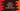# Python program to find the smallest divisor of a number## Smallest divisor of a number in Python :

This tutorial is to show you how we can find out the smallest divisor of a number in python. Number ‘y’ _is called divisor of number _‘x’ if ‘x/y’ is 0. Our program will ask the user to enter a no. It will then find out the lowest divisor of the number.

If the number is 10, then its divisors are 1,2,5 and 10. We will ignore 1 and consider 2 as its smallest divisor for the number.

### Python program :

Let’s try to implement it in python :

``````#1
num = int(input("Enter a number : "))

#2
for i in range(2, num+1):
#3
if num % i == 0:
print ("The smallest divisor for {} is {}".format(num, i))
break``````

### Explanation :

The commented numbers in the above program denote the step numbers below :

1. Ask the user to enter a number. Read it using input() function. It will read the user input data as a string. Convert it by wrapping it with int() function.
2. Run one for loop from 2 to the user input number.
3. For each number, check if we can divide the user input number by this number or not. We are using an_ if_ condition here. If the current number can divide the user input number, this will be the smallest divisor for that number. Print that number.

### Sample Outputs :

``````Enter a number : 13
The smallest divisor for 13 is 13

Enter a number : 14
The smallest divisor for 14 is 2

Enter a number : 100
The smallest divisor for 100 is 2``````

This program is available in Github.

### Conclusion :

We have learnt how to find out the smallest divisor of a number in python. Try to run the program and drop one comment below if you have any queries.